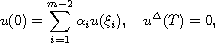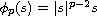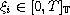Electron. J. Diff. Equ., Vol. 2014 (2014), No. 37, pp. 1-10.

### Existence of positive solutions for p-Laplacian an m-point boundary value problem involving the derivative on time scales Abdulkadir Dogan

Abstract:
We are interested in the existence of positive solutions for the -Laplacian dynamic equation on time scales,subject to the multipoint boundary condition,where,,,. By using fixed point theorems, we prove the existence of at least three non-negatvie solutions, two of them positive, to the above boundary value problem. The interesting point is the nonlinear term f is involved with the first order derivative explicitly. An example is given to illustrate the main result.

Submitted December 3, 2013. Published January 30, 2014.
Math Subject Classifications: 34B15, 34B16, 34B18, 39A10.
Key Words: Time scales; boundary value problem; p-Laplacian; positive solution; fixed point theorem.

Show me the PDF file (228 KB), TEX file, and other files for this article.Abdulkadir Dogan Department of Applied Mathematics Faculty of Computer Sciences Abdullah Gul University, Kayseri, 38039 Turkey Tel: +90 352 224 88 00 Fax: +90 352 338 88 28 email: abdulkadir.dogan@agu.edu.tr